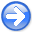PreconditioningNext: Balancing good data with Up: Preconditioning Previous: Need for an invertible

# INTERVAL VELOCITY

A bread-and-butter problem in seismology is building the velocity as a function of depth (or vertical travel time) starting from certain measurements. The measurements are described in many books, for example my book BEI (Basic Earth Imaging). They amount to measuring the integral of the velocity squared from the surface down to the reflector, known as the root-mean-square (RMS) velocity. Although good quality echoes may arrive often, they rarely arrive continuously for all depths. Good information is interspersed unpredictably with poor information. Luckily, we can also estimate the data quality by the coherency'' or the stack energy.'' In summary, what we get from observations and preprocessing are two functions of travel-time depth: (1) the integrated (from the surface) squared velocity, and (2) a measure of the quality of the integrated velocity measurement. Needed definitions are as follows:is a data vector in which components range over the vertical traveltime depth. Its component values contain the scaled RMS velocity squared, whereis the index on the time axis.is a diagonal matrix along which we lay the given measure of data quality. We use it as a weighting function.is the matrix of causal integration, a lower triangular matrix of ones.is the matrix of causal differentiation, namely,.is a vector containing the interval velocity squaredranging over the vertical traveltime depth.
From these definitions, under the assumption of a stratified Earth with horizontal reflectors (and no multiple reflections), the theoretical (squared) interval velocities enable us to define the theoretical (squared) RMS velocities by:(31)

In other words, any component ofmeasures the integral of a material property from the Earth surface to the depth of. We wish to find the material property everywhere, which is. If we integrate it from the surface downward with causal integration, we should get the measurements.

With imperfect data, our data fitting goal is to minimize the residual:(32)

whereis some weighting function, we need to choose. To find the interval velocity where there is no data (where the stack power theoretically vanishes), we have the model damping'' goal to minimize the wigglinessof the squared interval velocity.(33)

We precondition these two goals by changing the optimization variable from interval velocity squaredto its wiggliness. Substitutinggives the two goals expressed as a function of wiggliness.(34)(35)

SubsectionsPreconditioningNext: Balancing good data with Up: Preconditioning Previous: Need for an invertible

2015-05-07